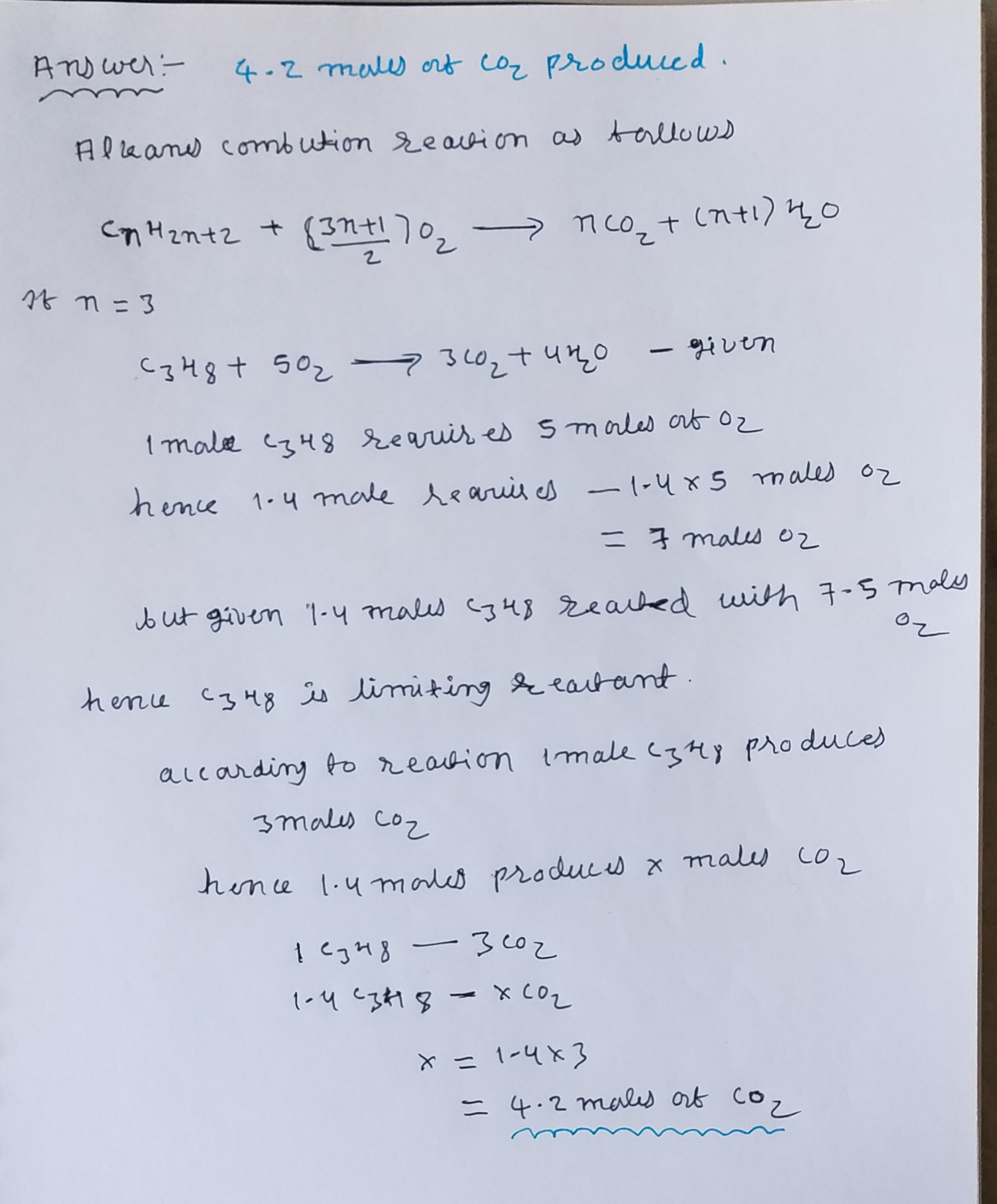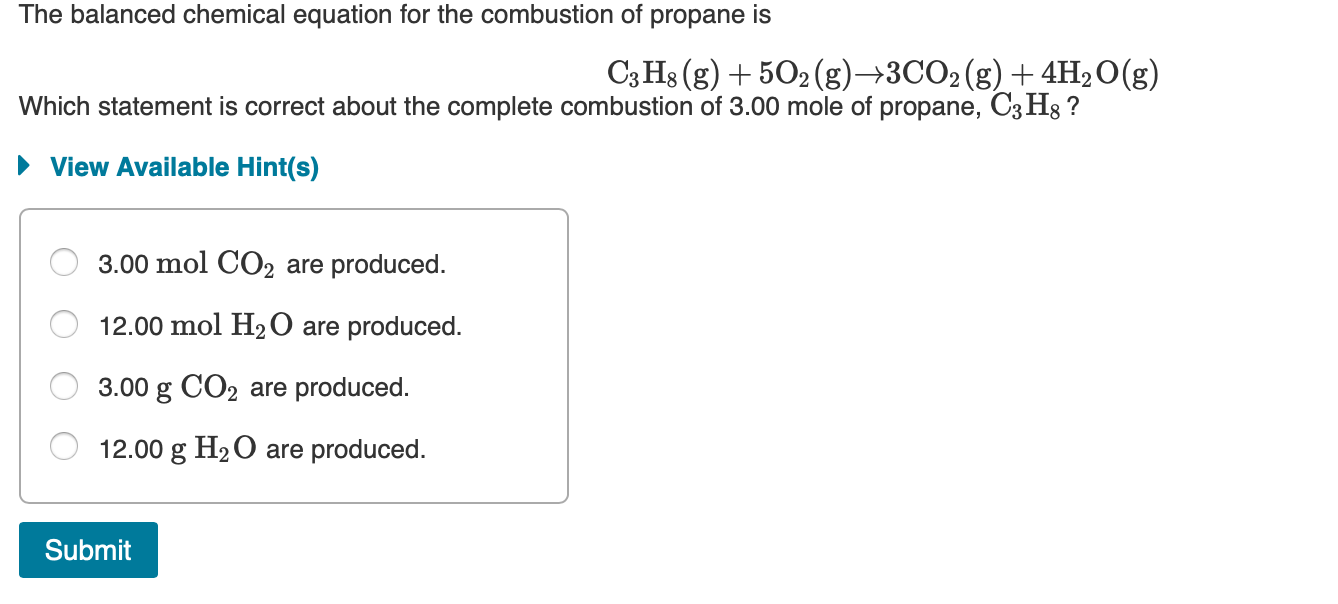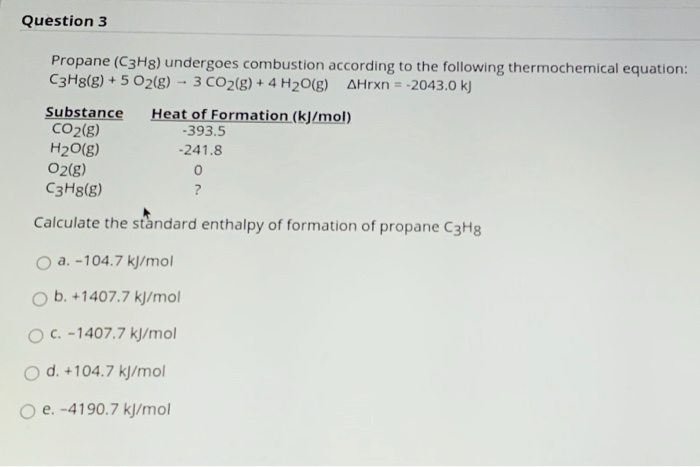Question

# The combustion of propane (C3H8) produces CO2 and H2O: C3H8 (g) + 5O2 (g) → 3CO2...

The combustion of propane (C3H8) produces CO2 and H2O: C3H8 (g) + 5O2 (g) → 3CO2 (g) + 4H2O (g) The reaction of 7.5 mol of O2 with 1.4 mol of C3H8 will produce ________ mol of CO2. Group of answer choices.#### Earn Coins

Coins can be redeemed for fabulous gifts.

Similar Homework Help Questions
• ### The combustion of propane, C3H8, occurs via the reaction C3H8(g)+5O2(g)→3CO2(g)+4H2O(g) with heat of formation values given...

The combustion of propane, C3H8, occurs via the reaction C3H8(g)+5O2(g)→3CO2(g)+4H2O(g) with heat of formation values given by the following table: Substance ΔH∘f (kJ/mol) C3H8 (g)=  -104.7 CO2(g)= −393.5 H2O(g)= −241.8 Calculate the enthalpy for the combustion of 1 mole of propane.

• ### The balanced chemical equation for the combustion of propane is C3H8(g) + 5O2(g) +3CO2(g) + 4H2O(g)...The balanced chemical equation for the combustion of propane is C3H8(g) + 5O2(g) +3CO2(g) + 4H2O(g) Which statement is correct about the complete combustion of 3.00 mole of propane, C3Hg ? ► View Available Hint(s) O 3.00 mol CO2 are produced. O 12.00 mol H2O are produced. O 3.00 g CO2 are produced. O 12.00 g H2O are produced. Submit

• ### The combustion of propane (C3H8) produces CO2 and H2O:

The combustion of propane (C3H8) produces CO2 and H2O: C3H3 (8) + 5 O2 (g) → 3 CO2 (g) + 4 H2O (g) mol The reaction of 2.5 mol of O2 with 4.6 mol of C3H8 will produce _______ of H2O. A) 4.0 B) 3.0 C) 2.5 D)2.0 E) 1.0

• ### calculate the enthalpy of the combustion of propane for your barbeque C3H8 + 5O2 -> 3CO2 + 4H2O propane -105 kJ...

calculate the enthalpy of the combustion of propane for your barbeque C3H8 + 5O2 -> 3CO2 + 4H2O propane -105 kJ/mol CO2 -394 kJ/mol Water -286 kJ/mol Ooxygen. 0

• ### Consider the combustion of propane: C3H8 (g) + 5O2 (g) → 3CO2 (g) + 4H2O(l) ΔH...

Consider the combustion of propane: C3H8 (g) + 5O2 (g) → 3CO2 (g) + 4H2O(l) ΔH = –2221 kJ Assume that all of the heat comes from the combustion of propane. Calculate ΔH in which 5.00 g of propane is burned in excess oxygen at constant pressure.

• ### The combustion of propane (C3H8) produces CO2 and H2O according to the following balanced equation: C3H8...

The combustion of propane (C3H8) produces CO2 and H2O according to the following balanced equation: C3H8 (g) + 5 O2 (g) → 3 CO2 (g) + 4 H2O (g) If the hydrocarbon is present in excess, what mass of oxygen (O2) in grams is necessary to form 12.9 g of CO2? Round your answer to the nearest 0.1.

• ### ﻿Propane (C3H8)burns according to the following balanced equation: C3H8(g)+5O2(g)→3CO2(g)+4H2O(g) Calculate ΔH∘rxnΔ for this reaction using standard...

﻿Propane (C3H8)burns according to the following balanced equation: C3H8(g)+5O2(g)→3CO2(g)+4H2O(g) Calculate ΔH∘rxnΔ for this reaction using standard enthalpies of formation. (The standard enthalpy of formation of gaseous propane is -103.9 kJ/molkJ/mol.) Express the enthalpy in kilojoules to four significant figures.

• ### Please help? Calculate deltaHr for the reaction: C3H8(g) + 5O2(g)----->3CO2(g) + 4H2O(l) Given: 3C(s) + 4H2(g)----->C3H8(g)...

Please help? Calculate deltaHr for the reaction: C3H8(g) + 5O2(g)----->3CO2(g) + 4H2O(l) Given: 3C(s) + 4H2(g)----->C3H8(g) deltaH -24.8 kcal    H2(g) + 1/2 O2(g)----->H2O(l) deltaH -68.3kcal C(s) + O2(g)-------> CO2(g) deltaH -94.0 kcal

• ### Combustion

The combustion of propane (C3H8) in thepresence of excess oxygen yields CO2 andH2OC3H8(g) + 5O2------------------> 3CO2 + 4H2OThe reaction of 2.5 mol of O2 willproduce-------------------mol of H2O

• ### Question 3 Propane (C3H8) undergoes combustion according to the following thermochemical equation: C3H8(g) + 5 O2(g)...Question 3 Propane (C3H8) undergoes combustion according to the following thermochemical equation: C3H8(g) + 5 O2(g) -- 3 CO2(g) + 4H2O(g) Arxn = -2043.0 kJ Substance Heat of Formation (kJ/mol) CO2(g) -393.5 H2O(g) -241.8 O2(g) 0 C3H8(g) ? Calculate the standard enthalpy of formation of propane C3H8 a. -104.7 kJ/mol ob. +1407.7 kJ/mol C. -1407.7 kJ/mol O d. +104.7 kJ/mol o e. -4190.7 kJ/mol